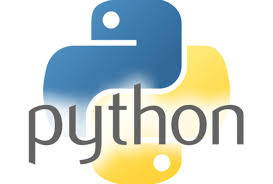31.How will you get all the keys from the dictionary?
Using dictionary.keys() function, we can get all the keys from the dictionary object.

print dict.keys() # Prints all the keys

32.How will you get all the values from the dictionary?
Using dictionary.values() function, we can get all the values from the dictionary object.

print dict.values() # Prints all the values

33.How will you convert a string to an int in python?
int(x [,base]) – Converts x to an integer. base specifies the base if x is a string.

34.How will you convert a string to a long in python?
long(x [,base] ) – Converts x to a long integer. base specifies the base if x is a string.

35.How will you convert a string to a float in python?
float(x) − Converts x to a floating-point number.

36.How will you convert a object to a string in python?
str(x) − Converts object x to a string representation.

37.How will you convert a object to a regular expression in python?
repr(x) − Converts object x to an expression string.

38.How will you convert a String to an object in python?
eval(str) − Evaluates a string and returns an object.

39.How will you convert a string to a tuple in python?
tuple(s) − Converts s to a tuple.

40.How will you convert a string to a list in python?
list(s) − Converts s to a list.